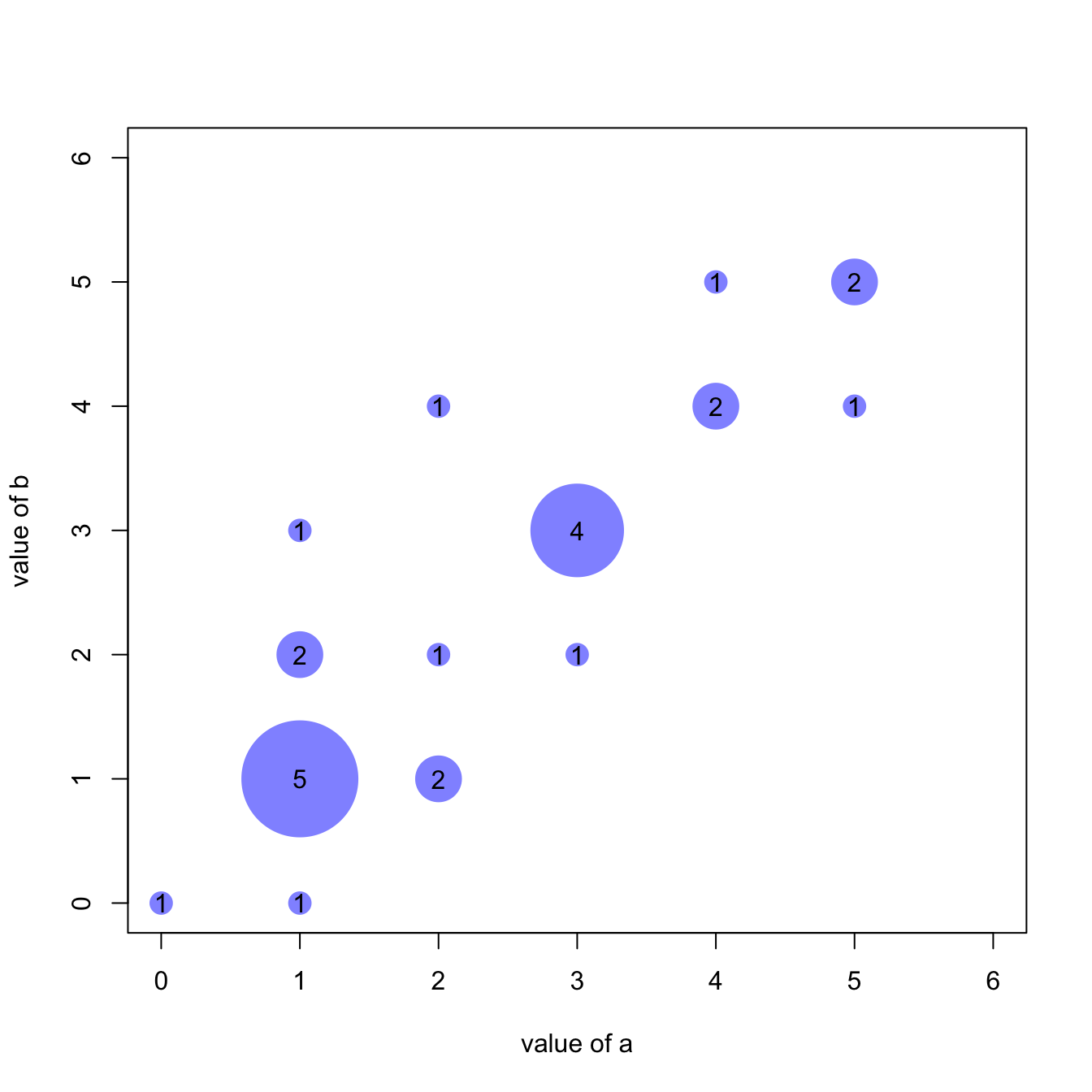# Correlation between discrete variable

Studying the relationship between 2 discrete variables is complicated since an usual scatterplot suffers overplotting. Here is a workaround using base R.

# Scatterplot with variable size

An usual scatterplot would suffer overplotting when used for discrete variables: dots would be drawn on top of each other, making the chart unreadable.

The workaround suggested here makes dot size proportional to the number of datapoints behind it. On top of that, the exact number can be represented in the bubble thanks to the `text()` function.``````#Let's create 2 discrete variables
a <- c(1,1,3,4,5,5,1,1,2,3,4,1,3,2,1,1,5,1,4,3,2,3,1,0,2)
b <- c(1,2,3,5,5,5,2,1,1,3,4,3,3,4,1,1,4,1,4,2,2,3,0,0,1)

#I count the occurence of each couple of values. Eg : number of time a=1 and b=1, number of time a=1 and b=2 etc...
AA <- xyTable(a,b)

#Now I can plot this ! I represent the dots as big as the couple occurs often
coeff_bigger <- 2
plot(AA\$x , AA\$y , cex=AA\$number*coeff_bigger  , pch=16 , col=rgb(0,0,1,0.5) , xlab= "value of a" , ylab="value of b" , xlim=c(0,6) , ylim=c(0,6) )
text(AA\$x , AA\$y , AA\$number )

#Note : It's easy to make a function that will compute this kind of plot automaticaly :
represent_discrete_variable <- function(var1, var2 , coeff_bigger){
AA=xyTable(var1,var2)
plot(AA\$x , AA\$y , cex=AA\$number*coeff_bigger  , pch=16 , col="chocolate1" , xlab= "value of a" , ylab="value of b" )
text (AA\$x , AA\$y , AA\$number )
}``````

# Other workarounds

Other workarounds could be considered in this situation:

Related chart types

## Contact

This document is a work by Yan Holtz. Any feedback is highly encouraged. You can fill an issue on Github, drop me a message on Twitter, or send an email pasting yan.holtz.data with gmail.com.